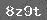6的乘法口诀

6的乘法口诀

（一）通过直观的实物图和乘法的含义，在老师的引导下，编出6的乘法口诀．

（二）找出6的乘法口诀的规律，初步熟记6的乘法口诀，会用乘法口诀正确求积．

（三）初步培养学生抽象概括能力．

（一）复习准备

1．复习25的乘法口诀

2．卡片口算，并说出用哪句口诀

2×3=　　　　　　　　 1×4=　　　　　　　　 2×1=　　　　　　　　　 5×2=

4×4=　　　　　　　　 5×5=　　　　　　　 　3×5=　　　　　　　　　 4×3=

3．卡片口算，直接记得数

2×5=　　　　　　　　　 2×2=　　　　　　　 5×1=　　　　　　　　　 3×4=

1×5=　　　　　　　　　 5×3=　　　　　　　 2×4=　　　　　　　　　 5×4=

（二）学习新课

1．准备练习36相加是多少？（18

56呢？（3066呢？（36

2．出示例11教师出示蝉图（图上共画6只蝉，第一次先露出1只，其它的蝉先用纸盖起来）．1）图上画的是什么？（1只蝉）

2）仔细数一数，一只蝉有几条腿？（1只蝉有6条腿）

316怎样用乘法算式表示？（学生回答后，教师在图的下面板书： 6×1=6

4　 6×1= 6这个算式表示什么意思？（一个六是六）

5）谁能结合乘法算式编一句乘法口诀？（一六得六）1 2只蝉共有多少条腿？怎样列式？（待学生回答后，教师板书： 6×2=12

2 6×2=12这个算式什么意思，谁能编出一句乘法口诀？（待学生回答后，教师在6×2=12算式旁边板书：二六十二）

6的乘法口诀前两句咱们已经编出来了，后面几句，同学们试着自己编好吗？

3．观察口诀，发现规律

16的乘法口诀有几句？（有6句）

2）怎样看出是6的乘法口诀？（每句口诀第二个字是六）

3）每句口诀第一个字表示什么？（几个6

4 6的乘法口诀的得数，后一句与前一句有什么关系？（后一句比前一句多6

5）如果你忘掉了其中的一句口诀，如四六（），你能不能用最快的方法想起它的得数？（小组讨论后再交流）

1）熟读口诀，自己试背口诀．

2）指名背，两人互相背．

3）师生对口令．

（三）巩固反馈

1．基本练习

1）你摆的六边形用了几根小棒？（6根）

2）摆1个六边形用6根小棒，如果不摆图了，你能知道摆2个六边形用几根小棒吗？摆3个，摆5个，摆6个呢？

2．发展性练习

1）先算出每道题的得数，再说一说每组两道题之间的关系．3）读一句口诀，说出两道乘法算式．

3．综合性练习

6×4=　　　　 1×6=　　　　　　　 4×6=　　　　　　 3×5=

2×6=　　　　 3×6=　　　　　　　 6×3=　　　　　　 4×3=相关推荐

 留言与评论（共有 0 条评论）

 验证码：